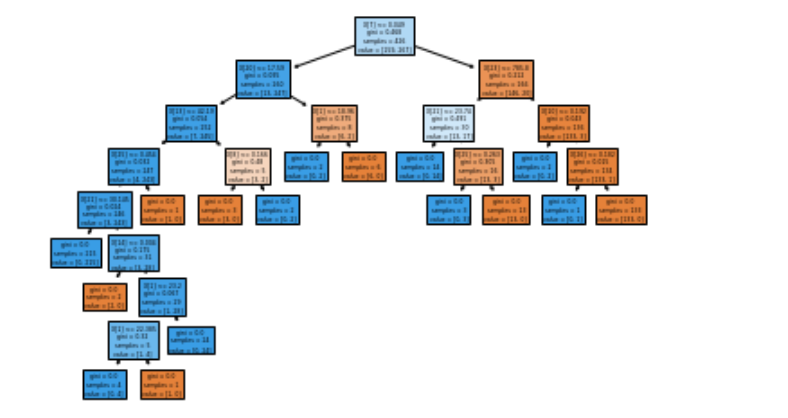# Sklearn: Tree diagram

The `plot_tree()` function allows you to create a diagram of steps present in a decision tree model:

``````import matplotlib.pyplot as plt
from sklearn import metrics, model_selection
from sklearn.tree import DecisionTreeClassifier, plot_tree

X_train, X_test, y_train, y_test = model_selection.train_test_split(X, y, random_state=0)
clf = DecisionTreeClassifier()
clf.fit(X_train, y_train)

plot_tree(clf, filled=True)
plt.show()
``````Tags:

Categories:

Updated: